Next: Charged Particle Motion in Up: Multi-Dimensional Motion Previous: Motion in a Two-Dimensional

Projectile Motion with Air Resistance

Suppose that a projectile of mass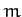is launched, at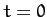, from ground level (in a flat plain), making an angle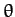to the horizontal. Suppose, further, that, in addition to the force of gravity, the projectile is subject to an air resistance force which acts in the opposite direction to its instantaneous direction of motion, and whose magnitude is directly proportional to its instantaneous speed. This is not a particularly accurate model of the drag force due to air resistance (the magnitude of the drag force is typically proportion to the square of the speed--see Section 3.3), but it does lead to tractable equations of motion. Hence, by using this model we can, at least, get some idea of how air resistance modifies projectile trajectories.

Let us adopt a Cartesian coordinate system whose origin coincides with the launch point, and whose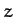-axis points vertically upward. Let the initial velocity of the projectile lie in the-plane. Note that, since neither gravity nor the drag force cause the projectile to move out of the-plane, we can effectively ignore thecoordinate in this problem.

The equation of motion of our projectile is written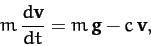(175)

where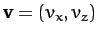is the projectile velocity,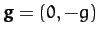the acceleration due to gravity, and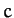a positive constant. In component form, the above equation becomes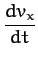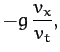(176)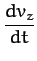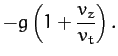(177)

Here,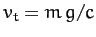is the terminal velocity: i.e., the velocity at which the drag force balances the gravitational force (for a projectile falling vertically downward).

Integrating Equation (176), we obtain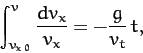(178)

where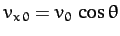is the-component of the launch velocity. Hence,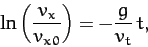(179)

or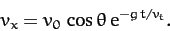(180)

It is clear, from the above equation, that air drag causes the projectile's horizontal velocity, which would otherwise be constant, to decay exponentially on a time-scale of order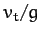.

Integrating Equation (177), we get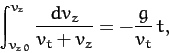(181)

where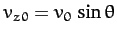is the-component of the launch velocity. Hence,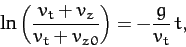(182)

or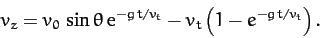(183)

It thus follows, from Equations (180) and (183), that if the projectile stays in the air much longer than a time of orderthen it ends up falling vertically downward at the terminal velocity,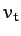, irrespective of its initial launch angle.

Integration of (180) yields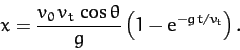(184)

In the limit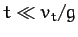, the above equation reduces to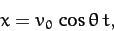(185)

which is the standard result in the absence of air drag. In the opposite limit,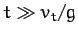, we get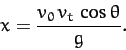(186)

The above expression clearly sets an effective upper limit on how far the projectile can travel in the horizontal direction.

Integration of (183) gives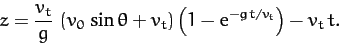(187)

In the limit, this equation reduces to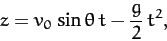(188)

which is the standard result in the absence of air drag. In the opposite limit,, we get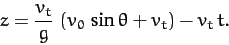(189)

Incidentally, the above analysis implies that air resistance only starts to have an appreciable effect on the trajectory after the projectile has been in the air a time of order.

It is clear, from the previous two equations, that the time of flight of the projectile (i.e., the time at which, excluding the trivial result) is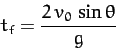(190)

when, which implies that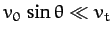, and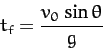(191)

when, which implies that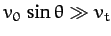(i.e., the vertical component of the launch velocity is much greater than the terminal velocity). It thus follows, from Equations (185) and (186), that the horizontal range [i.e.,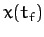] of the projectile is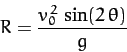(192)

when, and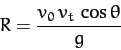(193)

when. Equation (192) is, of course, the standard result without air resistance. This result implies that, in the absence of air resistance, the maximum horizontal range,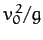, is achieved when the launch angletakes the value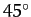. On the other hand, Equation (193) implies that, in the presence of air resistance, the maximum horizontal range,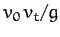, is achieved whenis made as small as possible. However,cannot be made too small, since expression (193) is only valid when. In fact, assuming that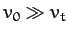, the maximum horizontal range,, is achieved when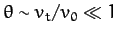. We thus conclude that if air resistance is significant then it causes the horizontal range of the projectile to scale linearly, rather than quadratically, with the launch velocity,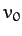. Moreover, the maximum horizontal range is achieved with a launch angle which is much shallower than the standard result,.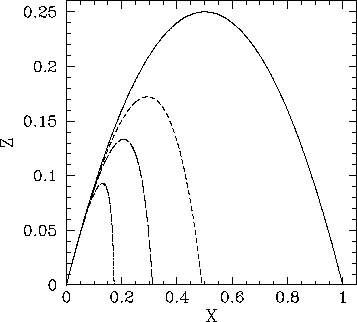Figure 11 shows some example trajectories calculated, from the above model, with the same launch angle,, but with different values of the ratio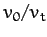. Here,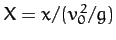and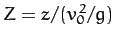. The solid, short-dashed, long-dashed, and dot-dashed curves correspond to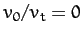,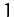,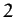, and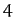, respectively. It can be seen that as the air resistance strength increases (i.e., asincreases), the range of the projectile decreases. Furthermore, there is always an initial time interval during which the trajectory is identical to that calculated in the absence of air resistance (i.e.,). Finally, in the presence of air resistance, the projectile tends to fall more steeply than it rises. Indeed, in the presence of strong air resistance (i.e.,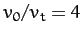), the projectile falls almost vertically.Next: Charged Particle Motion in Up: Multi-Dimensional Motion Previous: Motion in a Two-Dimensional
Richard Fitzpatrick 2011-03-31